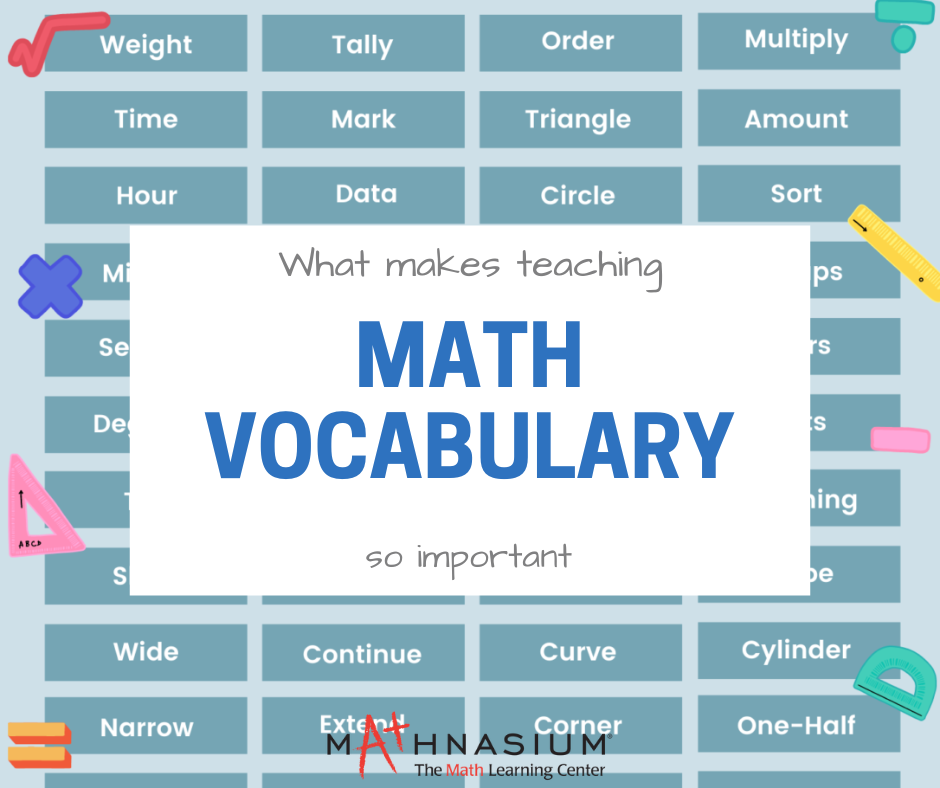877-601-6284
Get Started by Finding a Local Center

# Why Math Vocabulary is Important

Apr 25, 2023 | Happy ValleyMathematics is a universal language we use to understand and make sense of the world. It is a language that helps us describe and explain the most intricate concepts in science, engineering, and technology. However, it is not only the concepts that matter when it comes to understanding math but also the vocabulary. We cannot fully grasp and communicate mathematical concepts without the right vocabulary. This is why teaching math vocabulary is vital. In this blog, we'll explore the reasons why.

• Understanding Mathematical Concepts Mathematical concepts are complex, and they require a lot of abstract thinking. To understand them, students need to know the specific terminology used in math. Vocabulary words like "fraction," "integer," and "polygon" are just a few examples of essential math terms that students need to know. Without this knowledge, anyone would struggle to understand even the simplest of math problems.

• Communicating Mathematical Ideas Mathematical vocabulary is the key to communicating mathematical ideas. When you know the right vocabulary, you can explain thought processes and reasoning behind solving a math problem. Naturally, it is easier for communicate ideas when we have the right words to use. This is essential for success in every field and of course is true if you want to build a strong foundation in mathematics.

• Building Mathematical Confidence When we understand math vocabulary, we feel more confident in our ability to solve math problems. The knowledge of math terms and concepts empowers students to solve complex math problems and understand the logic behind mathematical operations. When you feel confident in your abilities, you are more likely to engage in the learning process and feel a sense of pride in your accomplishments.

• Preparing for Standardized Tests As you know, standardized tests such as the SAT, ACT, and GRE all include math sections. These tests often use complex math vocabulary, and without knowing the right terminology, it is easy for students to struggle to understand the questions. Math vocabulary, is a vital foundation to have and can impact a person’s future academic and career opportunities.

• Enhancing Critical Thinking Skills Mathematics is a subject that requires critical thinking skills. It is not enough to memorize formulas and procedures; students must be able to understand how and why they work. By teaching math vocabulary, educators can help students build critical thinking skills by requiring them to analyze and understand the meanings behind mathematical terms.

Math vocabulary is a crucial component of mathematics education. Understanding math vocabulary is essential for understanding concepts, communicating ideas, building confidence, preparing for standardized tests, and enhancing critical thinking skills. By prioritizing math vocabulary instruction, we can help students build a strong foundation in mathematics and set them up for success in future academic and career endeavors.

##### Locations near
Could not find Center, try again